swtch.com Go back Open original

# An Encoded Tree Traversal Posted on Monday, February 25, 2019. PDF

By Russ Cox

Every basic data structures course identifies three ways to traverse a binary tree. It’s not entirely clear how to generalize them to k-ary trees, and I recently noticed an unexpected ordering that I’d like to know more about. If you know of references to this ordering, please leave a comment or email me (rsc@swtch.com).

First a refresher about binary-tree orderings to set up an analogy to k-ary trees.

Preorder visits a node before its left and right subtrees: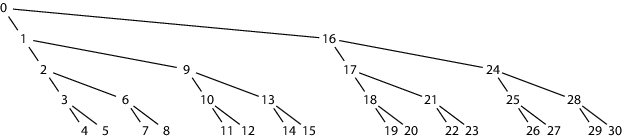Inorder visits a node between its left and right subtrees: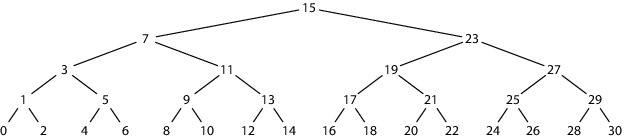Postorder visits a node after its left and right subtrees: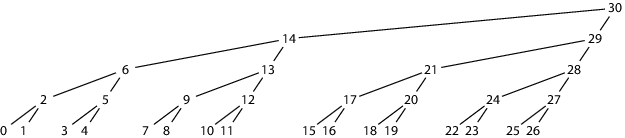Each picture shows the same 16-leaf, 31-node binary tree, with the nodes numbered and also placed horizontally using the order visited in the given traversal.

It was observed long ago that one way to represent a tree in linear storage is to record the nodes in a fixed order (such as one of these), along with a separate array giving the number of children of each node. In the pictures, the trees are complete, balanced trees, so the number of children of each node can be derived from the number of total leaves. (For more, see Knuth Volume 1 §2.3.1; for references, see §2.3.1.6, and §2.6.)

It is convenient to refer to nodes in a tree by a two-dimensional coordinate (l, n), consisting of the level of the node (with 0 being the leaves) and its sequence number at that level. For example, the root of the 16-node tree has coordinate (4, 0), while the leaves are (0, 0) through (0, 15).

When storing a tree using a linearized ordering such as these, it is often necessary to be able to convert a two-dimensional coordinate to its index in the linear ordering. For example, the right child of the root—node (3, 1)—has number 16, 23, and 29 in the three different orderings.

The linearized pre-ordering of (l, n) is given by:

seq(L, 0) = 0 (L is height of tree)
seq(l, n) = seq(l+1, n/2) + 1 (n even)
seq(l, n) = seq(l+1, n/2) + 2l+1 (n odd)

This ordering is awkward because it changes depending on the height of the tree.

The linearized post-ordering of (l, n) is given by:

seq(0, n) = n + n/2 + n/4 + n/8 + ...
seq(l, n) = seq(l–1, 2 n) + 1 = seq(0, 2l n) + l

This ordering is independent of the height of the tree, but the leaf numbering is still a little complex.

The linearized in-ordering is much more regular. It’s clear just looking at it that seq(0, n) = 2 n, and in fact a single equation applies to all levels:

seq(l, n) = 2l+1 n + 2l – 1

If you need to linearize a complete binary tree, using the in-order traversal has the simplest math.

The three binary orderings correspond to visiting the node after 0, 1, or 2 of its child subtrees. To generalize to k-ary trees, we can visit the node after any number of subtrees from 0 to k, producing k+1 orderings. For example, for k = 3, here are the four generalized orderings of a 27-leaf, 39-node 3-ary tree:

Preorder (inorder-0):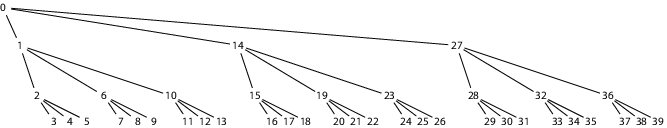Inorder-1: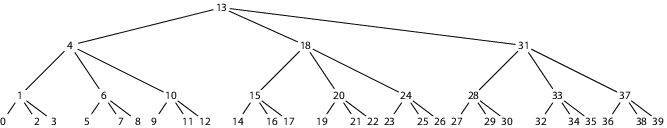Inorder-2: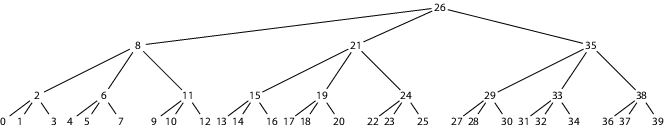Postorder (inorder-3):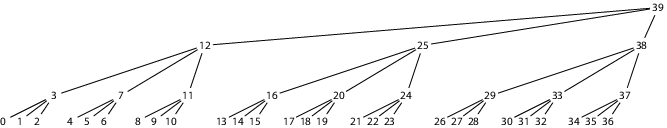Just looking at the leaves of each, none of them has a nice simple form with regular gaps like the seq(0, n) = 2 n of in-order traversal for binary trees. Instead, both the possible “in-order” traversals end up with equations more like the post-order traversal. What happened? Where did the nice, regular pattern go?

In a binary tree, the in-order numbering has the property that after the first leaf, one new parent (non-leaf) node is introduced before each additional one leaf. This works out nicely because the number of parent nodes in a binary tree of N leaves is N–1. The number of parents nodes in a k-ary tree of N leaves is (N–1)/(k–1), so we could try to build a nicer numbering by, after the first leaf, introducing one new parent node before each additional k–1 leaf nodes. That is, the leaves would be numbered by

seq(0, n) = n + (n+k–2)/(k–1),

which gives this leaf structure: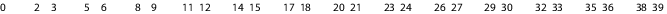But how do we fill in the gaps? The first three triples—0, 2, 3 and 5, 6, 8 and 9, 11, 12—clearly get nodes 1, 4, 7, and 10 as their three parents and one grandparent, but which is the grandparent? The binary in-order traversal was very self-similar, so let’s try the same thing here: after the first node, reserve one node for higher levels before each k–1 nodes at this level. That is, the parents are 1, 7, 10, and the grandparent is 4.

Applying this process throughout the tree, we end up with this traversal order (inorder-G, for gap-induced):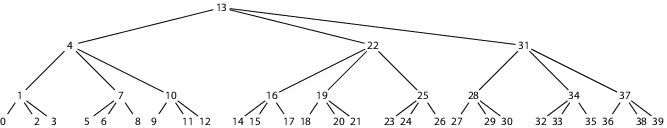For contrast, here is the ordering from the previous section that visited each node after its first subtree (inorder-1):The inorder-1 traversal has a regular tree structure but irregular numbering. In contrast, the inorder-G traversal has an irregular tree structure but very regular numbering that generalizes the binary inorder numbering:

seq(l, n) = kl (n+k–2)/(k–1) + kl-1 + kl-2 + ... + k0

For a binary tree, inorder-1 and inorder-G are the same: the traversal has both a regular tree structure and a regular numbering. But for k-ary trees, it seems you can pick only one.

The regularity of the numbering is easiest to see in base k. For example, here is the binary inorder traversal with binary numbering: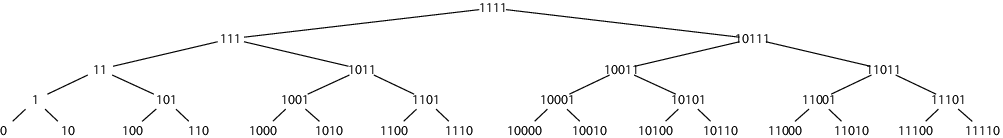The bottom row uses numbers ending in 0; the next row up uses numbers ending in 01; then 011; and so on.

For the 3-ary tree, it is the inorder-G traversal (not inorder-1 or inorder-2) that produces an equivalent pattern:The bottom row uses numbers ending in 0 or 2; the next row up uses numbers ending in 01 or 21; then 011 or 211; and so on.

Through a roundabout way, then, we’ve ended up with a tree traversal that’s really just a nice base-k encoding. There must be existing uses of this encoding, but I’ve been unable to find any or even determine what its name is.

Does anyone know of any places this has appeared before? I’d love to read about them. Thanks.